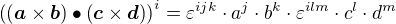## Cross Product

The definition of the cross product is:... whereis the Levi-Civita Symbol.

### Where does the angle come from?

First, there is an identity connecting the cross product to the scalar product:So the identity is:If the vectors c and a are the same and the vectors d and b are the same, it follows:Hence the angle in the cross product comes from the angle in the scalar product:

## Scalar Product

Given a triangle with sides a and b and c=b-a, the Law of Cosines says:On the other hand, using only the scalar product distributivity:So since, it follows that: